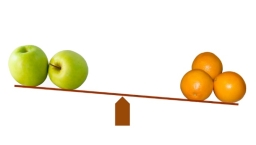Which 6

Which statement is correct about the product of 423 and 78?

A. Because 78 is greater than 1, the product of 423 and 78 is greater than 423.
B. Because 78 is less than 1, the product of 423 and 78 is greater than 423.
C. Because 78 is greater than 1, the product of 423 and 78 is less than 423.
D. Because 78 is less than 1, the product of 423 and 78 is less than 423.

Result

x = (Correct answer is: A)Step-by-step explanation:Did you find an error or inaccuracy? Feel free to write us. Thank you!